CBSE Class 12 Sample Paper for 2018 Boards

Class 12
Solutions of Sample Papers and Past Year Papers - for Class 12 Boards

### If y = log (√x + 1 / √x) 2  then prove that x (x + 1) 2 y 2 + (x + 1) 2 y 1 = 2

This is a question of CBSE Sample Paper - Class 12 - 2017/18.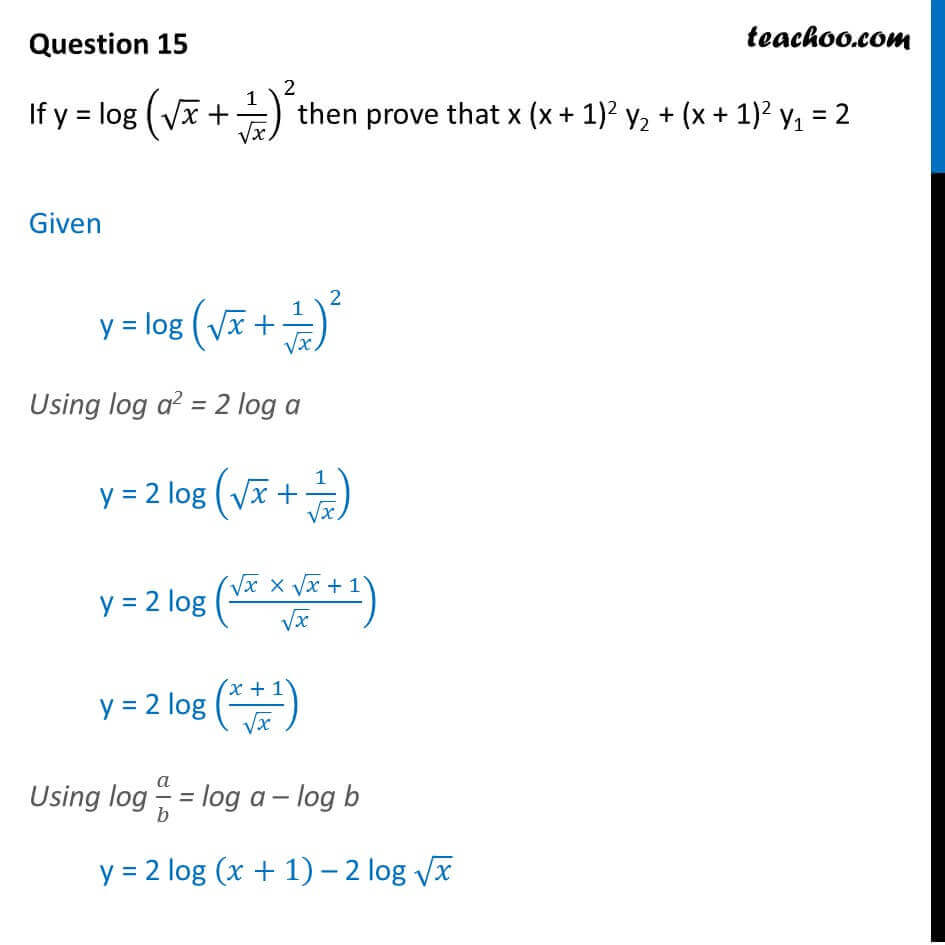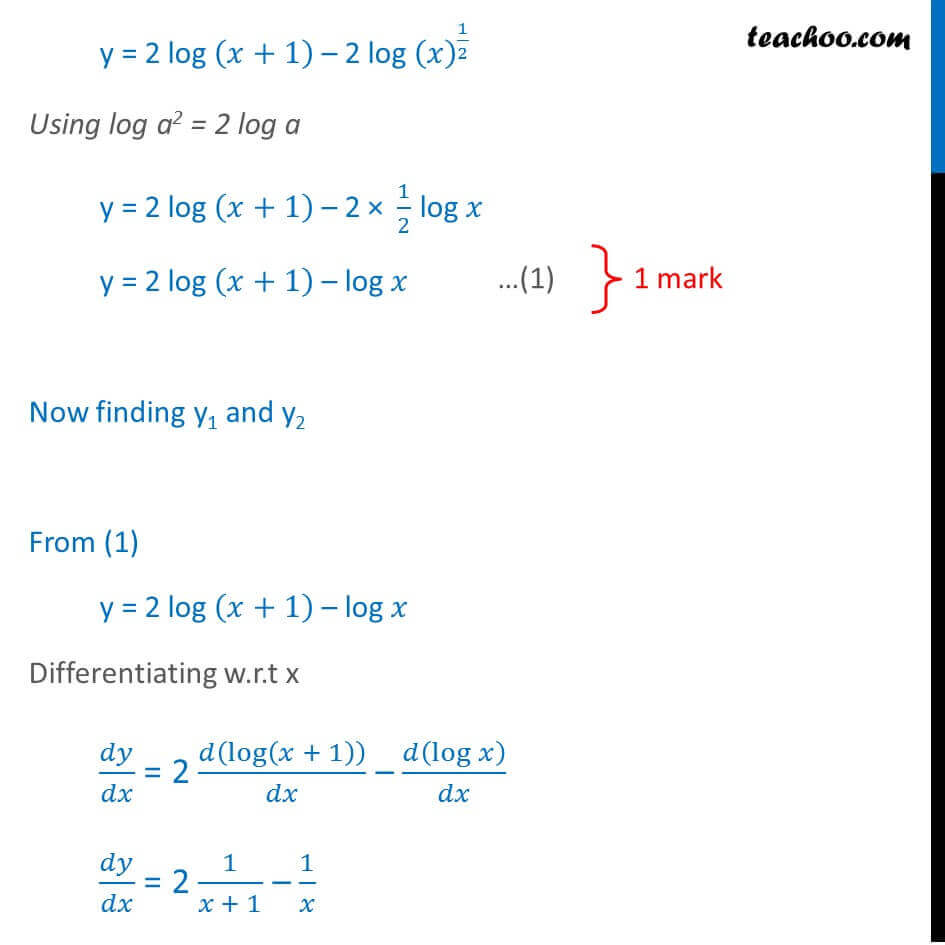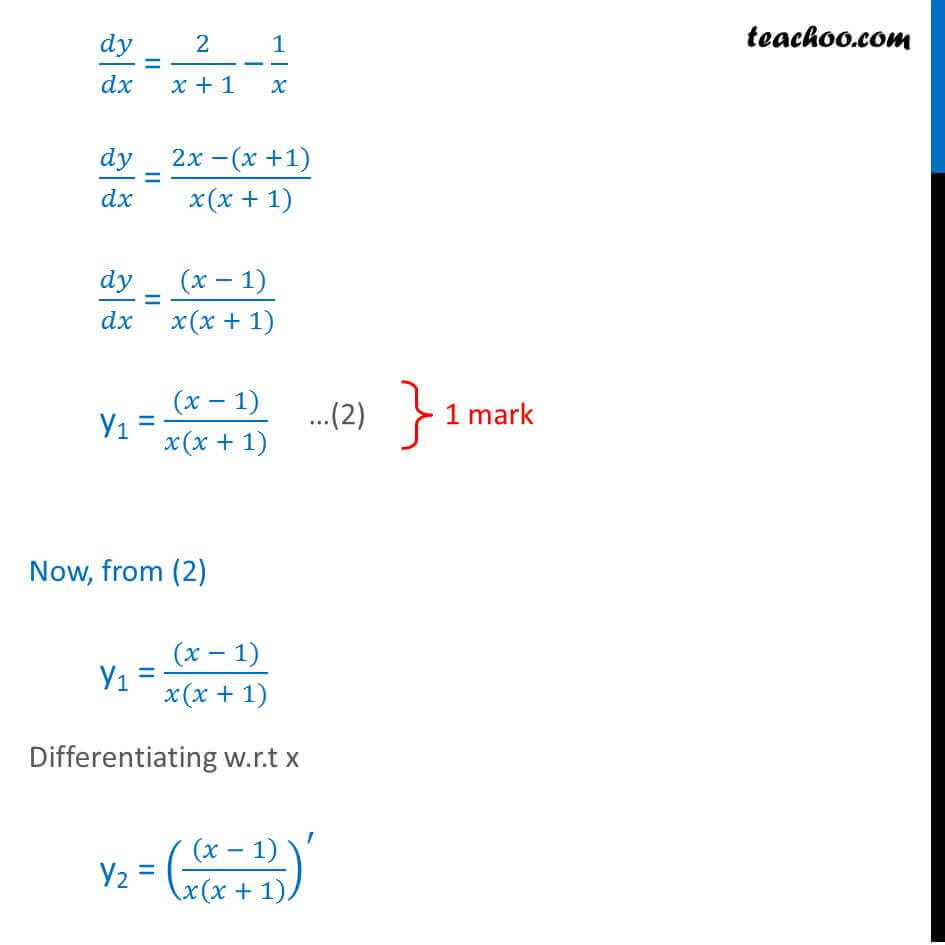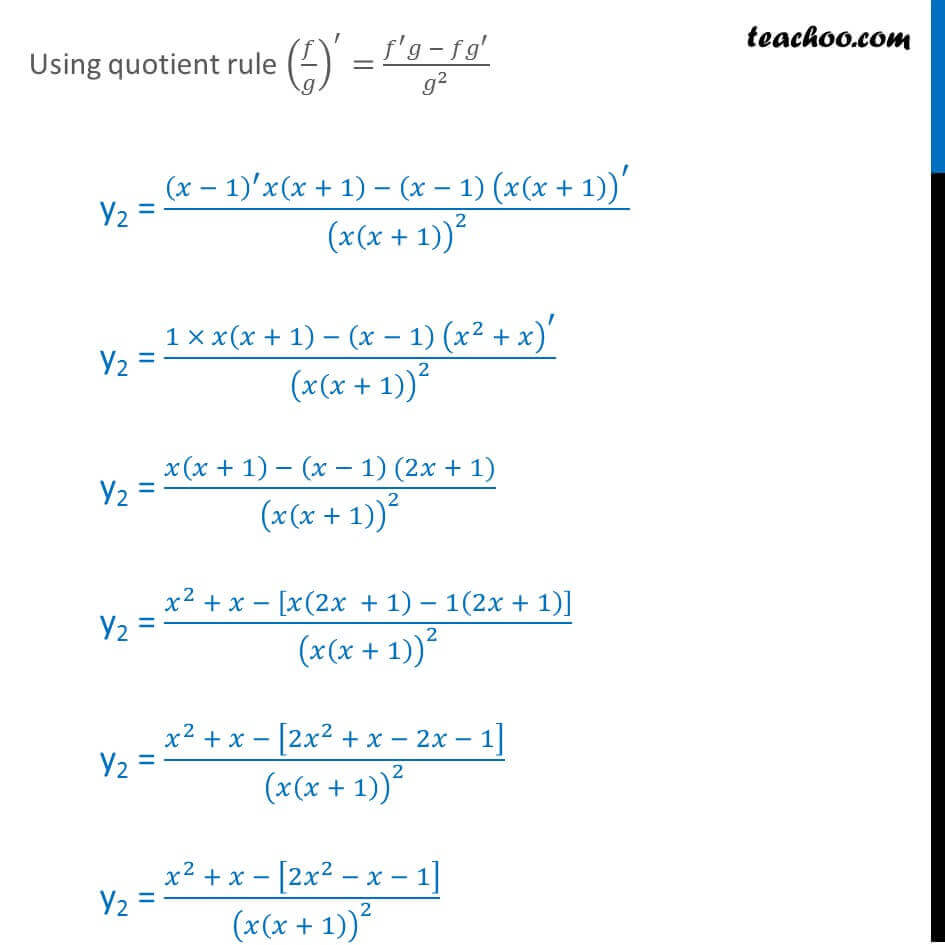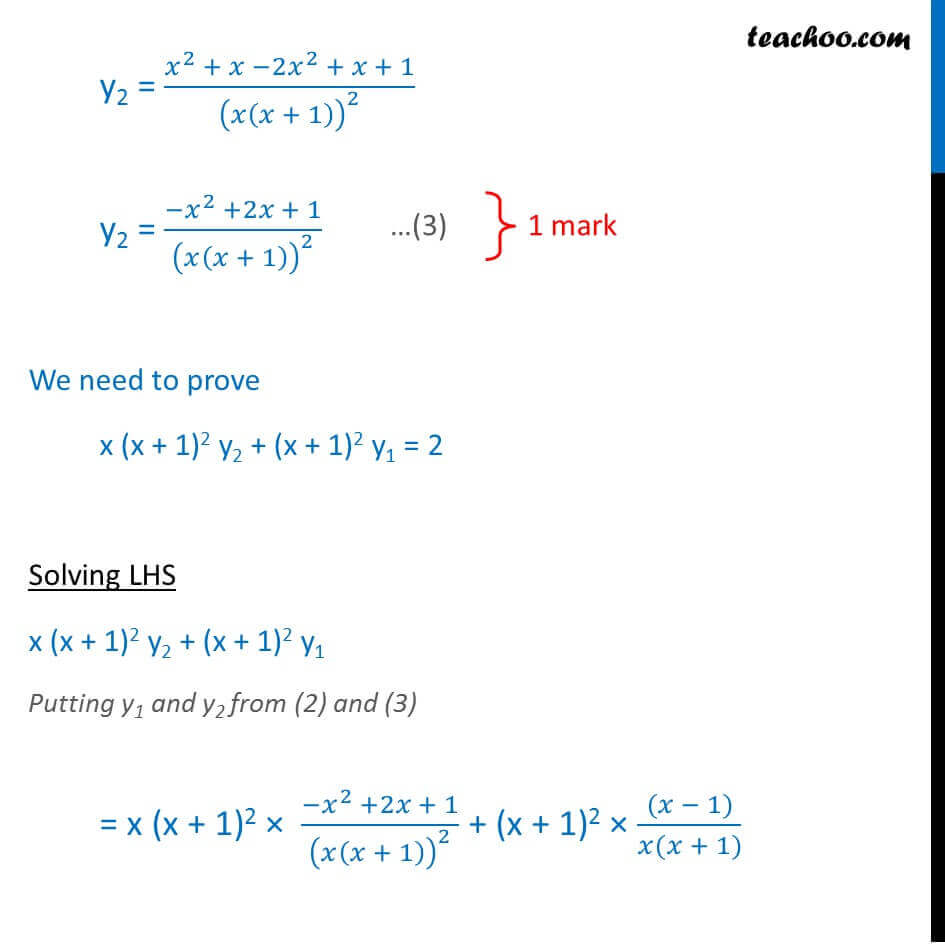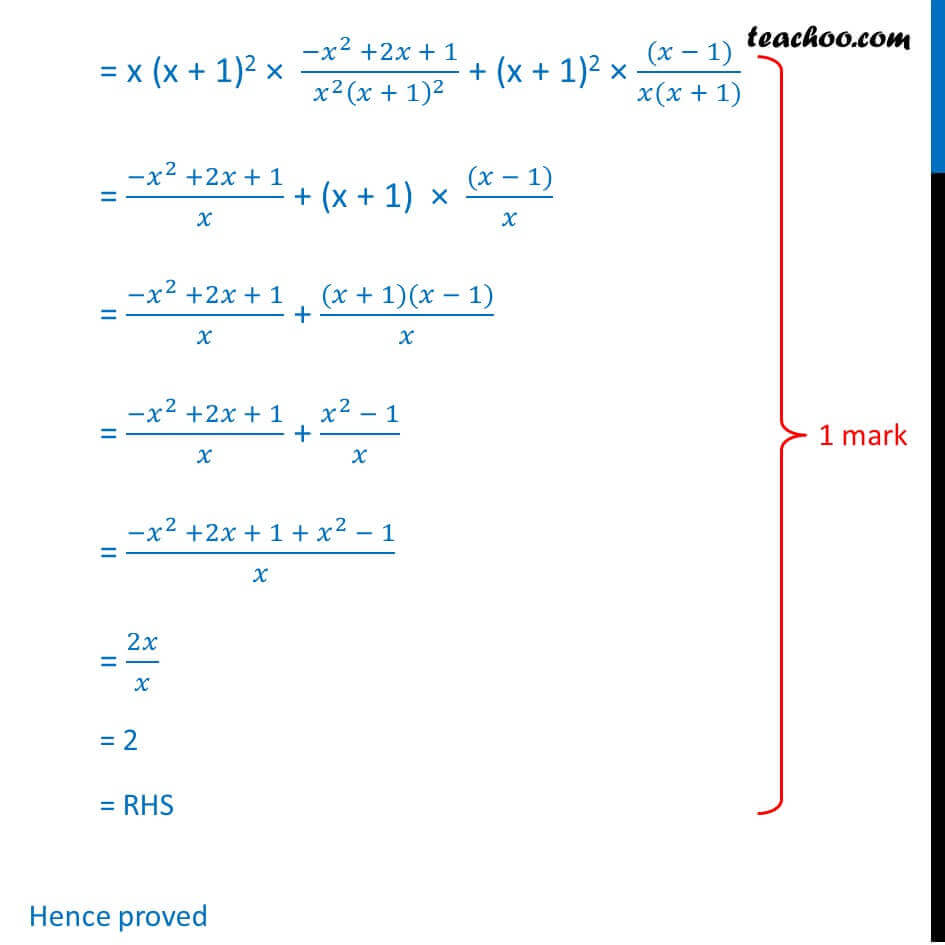Get live Maths 1-on-1 Classs - Class 6 to 12

### Transcript

Question 15 If y = log ( +1/ )^2then prove that x (x + 1)2 y2 + (x + 1)2 y1 = 2 Given y = log ( +1/ )^2 Using log a2 = 2 log a y = 2 log ( +1/ ) y = 2 log (( + 1)/ ) y = 2 log (( + 1)/ ) Using log / = log a log b y = 2 log ( +1) 2 log y = 2 log ( +1) 2 log ( )^(1/2) Using log a2 = 2 log a y = 2 log ( +1) 2 1/2 log y = 2 log ( +1) log Now finding y1 and y2 From (1) y = 2 log ( +1) log Differentiating w.r.t x / = 2 (log ( + 1) )/ (log )/ / = 2 1/( + 1) 1/ / = 2/( + 1) 1/ / = (2 ( +1))/( ( + 1)) / = (( 1))/( ( + 1)) y1 = (( 1))/( ( + 1)) Now, from (2) y1 = (( 1))/( ( + 1)) Differentiating w.r.t x y2 = ((( 1))/ ( + 1) )^ = x (x + 1)2 ( ^2 +2 + 1)/( ^2 ( + 1)^2 ) + (x + 1)2 (( 1))/( ( + 1)) = ( ^2 +2 + 1)/ + (x + 1) (( 1))/ = ( ^2 +2 + 1)/ + (( + 1)( 1))/ = ( ^2 +2 + 1)/ + ( ^2 1)/ = ( ^2 +2 + 1 + ^2 1)/ = 2 / = 2 = RHS Hence proved